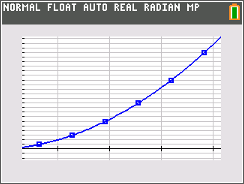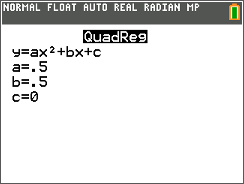•• ##### Device
• TI-84 Plus
•TI-84 Plus CE

# SA and NT: Stacking Bricks

by Texas Instruments#### Overview

This activity presents a real-world situation--stacking bricks in a pile--that can be modeled by a polynomial function. Students create a small table to show how the number of bricks relates to the number of rows, and calculate the first, second, and third differences of the data. Next they use the graphing calculator's statistical calculation functions to perform the correct regression. Finally, they evaluate the model using a variety of methods.

#### Key Steps

•Students start by determining the number of bricks in a stack with 1, 2, 3, 4, 5, and 6 rows. Then they will calculate the successive differences and record the values in the table on the worksheet. Students also need to enter the data for L1 and L2 in the calculator.

•Now students should use the QuadReg command from the Calc menu. Explain to students that when they enter L1, L2, Y1 after the command, this tells the calculator that the x-values they want to model are in L1, the y-values are in L2, and the equation for the model should be stored in Y1.

•Students will now compare the model to the data by creating a scatter plot. The graph screen should appear as shown at the right with the line passing through all of the points.